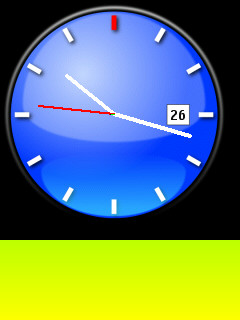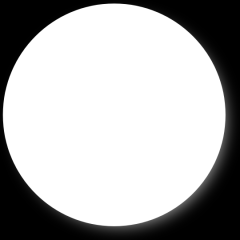### Unleash the power of PNG transparency with masks

Although PyS60 is not able to deal with images with alpha information (32-bit or RGBA), there is one way to show transparent GIF and PNG images using the mask parameter on Image class.

Suppose you have drawn a very cool clock with Inkscape having a transparent background and that you have exported it to PNG and would like to overlay this PNG on a gradient (green to yellow) background.

The first natural move would be the following code (supposing you have the image files on e:\python\lib\ folder):

```self.gradient = Image.open(u'e:\\python\\lib\\gradient.png') self.clock_img = Image.open(u'e:\\python\\lib\\clock.png') self.canvas.clear() self.canvas.blit(self.gradient) self.canvas.blit(self.clock_img)```

But the result isn’t what you would like to see:Transparent background are converted to a flat color.

To solve this problem there’s a mask parameter on blit method to help graphics to know where the transparent pixels on image are.

The mask can be an 1-bit image (black and white) or 8-bit image (grayscale). Blit method doesn’t accept other image modes on mask image (12, 16 or 24 bits).

If you search at Forum Nokia’s Wiki, you’ll find an example of an automask function, that automatically creates an image mask from another image, using the first pixel as transparent color.

```def automask(im):     width, height = im.size        # get image size     mask = Image.new(im.size, '1') # black and white     tran = im.getpixel((0,0))   # transparent top-left     for y in range(height):         line = im.getpixel([(x, y) for x in range(width)])         for x in range(width):             if line[x] == tran:                 mask.point((x,y), 0)  # mask on the point     return mask```

This function creates a mask image to be used on blit method as shown bellow:

```self.gradient = Image.open(u'e:\\python\\lib\\gradient.png') self.clock_img = Image.open(u'e:\\python\\lib\\clock.png') self.mask = automask(self.clock_img) self.canvas.clear() self.canvas.blit(self.gradient) self.canvas.blit(self.clock_img, mask=self.mask)```

The result of applying this function is thousand times better than the black background replacing transparent pixels, but it doesn’t support anti-alias and partially transparent pixels.

The result is shown bellow (note the absence of anti-alias on clock boundaries):The solution for the anti-alias and partially transparent pixels is a mask with 8-bit depth, where black is 100% transparent and white is 0% transparent. The grayscale gives the percentual of opacity of each pixel.

Using your favorite image processing program (BTW, mine is Gimp), you can extract only the alpha channel and export it as grayscale. You’ll have to invert the image to have white instead of black and vice-versa, like the image bellow.With this image mask, you can use the code bellow to apply the mask and use the PNG transparency:

```self.gradient = Image.open(u'e:\\python\\lib\\gradient.png') self.clock_img = Image.open(u'e:\\python\\lib\\clock.png') mask = Image.open(u'e:\\python\\lib\\clock-mask.png') #temporary mask self.mask = Image.new(mask.size, 'L') #create the mask with 8-bits grayscale mode self.mask.blit(mask) #copy temp mask to grayscale mask self.canvas.clear() self.canvas.blit(self.gradient) self.canvas.blit(self.clock_img, mask=self.mask)```

The result is shown bellow: